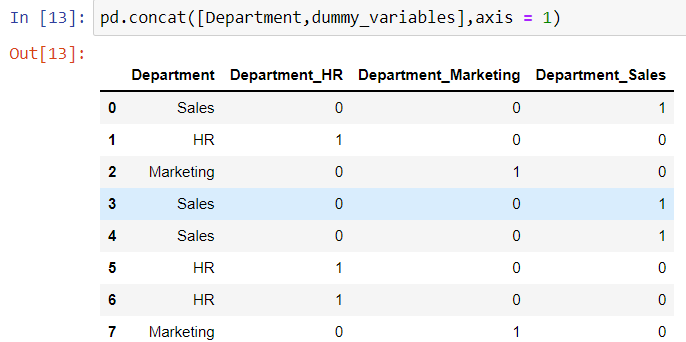top of page
Search
• Ekta Aggarwal

# One hot encoding and label encoding in Python

While dealing with data often there are categorical columns which can be nominal or ordinal in nature. Thus, to make best use of them we need to convert them to numbers to make better sense. In this article we shall be comparing 2 approaches of modifying our categorical variables:

Let us firstly load the necessary libraries for this tutorial:

```import pandas as pd
from sklearn.preprocessing import OneHotEncoder
from sklearn.preprocessing import LabelEncoder```

### One Hot Encoding (Dummy Variables)

Let us consider the data where we have employee information and the department they are working for. Since department is a categorical variable thus we can denote them in a 1 - 0 format.

Suppose we have 3 departments: HR, Sales and Marketing. We can create 3 columns for each of them namely: Department_HR, Department_Sales and Department_Marketing.When the department is HR then Department_HR= 1 and other 2 variables are 0. Similarly for sales department Department_Sales = 1 and other 2 will be 0. Lastly, for marketing department Department_Marketing= 1 and others as 0.

Note: It can never happen that there can be more than one 1 in a set of dummy variables. In a single row for dummy variables there can be at most one 1.

When categorical variables can be expressed in 1-0 notation - these are called dummy variables.

For one hot encoding we can use sklearn's OneHotEncoder( ) and pandas' get_dummies( ) function

```# Creating a dataframe
Department = ['Sales','HR','Marketing','Sales','Sales','HR','HR','Marketing']
Department = pd.DataFrame(Department, columns=['Department'])```##### Method 1: Using sklearn's OneHotEncoder

We load our OneHotEncoder( ) in an object named 'oh' . Then we fit our one hot encoder for our dataframe 'Department' . Note to see the output of dummy variables we have firstly converted the object to array using toarray( ) and then finally converted it to a data frame.

```oh = OneHotEncoder()
Department_dummies = oh.fit_transform(Department).toarray()
Department_dummies = pd.DataFrame(Department_dummies)```##### Method 2: Using pandas' get_dummies( )

Using pandas' get_dummies( ) function we can create dummy variables with a single line of code.

It is to be notes that get_dummies( ) only creates dummy variables. To append it in our data we use pandas' concat function:

Let us firstly save our dummy variables in a dataset.

`dummy_variables = pd.get_dummies(data=Department)`

We now concatenate our original data using pd.concat( ) , by defining axis = 1 or axis = "columns" we are telling Python to add the columns horizontally (and not append them as rows).

```pd.concat([Department,dummy_variables],axis = 1)

#alternatively
pd.concat([Department,dummy_variables],axis = "columns")```When Department_HR = 1 and Department_Marketing is 0 then it is self-implied that Department_Sales will be 0. Thus in this case we only need 3-1 = 2 dummy variables. We can drop the first column by specifying drop_first = True.

`catDf = pd.get_dummies(data=Department,drop_first=True)`

##### Label Encoding

When the categorical variables are ordinal in nature (Eg., “extremely dislike”, “dislike”, “neutral”, “like”, “extremely like” ) where extremely dislike has a least impact and extremely like has highest impact, i.e., you can order the categories; in such a situation you can use label encoding instead of one hot encoding.

For instance, we shall consider the list named Status:

```Status = ['High','High','Medium','Medium','Low','Low']
Status = pd.DataFrame(Status, columns= ['Status'])````le = LabelEncoder()`

Fitting the label encoder on our dataframe Status

`X_2 = le.fit_transform(Status)`

Output:

array([0, 0, 2, 2, 1, 1])

inverse_transform

Using inverse_transform we can retrieve our original labels of High, Medium and Low.

`le.inverse_transform(X_2)`

Output:

array(['High', 'High', 'Medium', 'Medium', 'Low', 'Low'], dtype=object)

Food for thought!

Label Encoder by default provides the labels in ascending order. To manually set the labels we can use index function.

index( ) function returns the index of the element in a list. In the following example, Low has index 0, Medium has index 1, High has index 2. Using lambda function, each element is being passed and corresponding index for that element is returned as an output.

We can use the following labelling to indicate High should have highest label and Low should have least.

`new_order = Status['Status'].apply(lambda x: ['Low', 'Medium', 'High'].index(x))`bottom of page